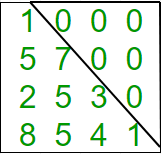GeeksforGeeks App
Open AppBrowser
Continue

# C++ Program to check if matrix is lower triangular

Given a square matrix and the task is to check the matrix is in lower triangular form or not. A square matrix is called lower triangular if all the entries above the main diagonal are zero.Examples:

```Input : mat = {{1, 0, 0, 0},
{1, 4, 0, 0},
{4, 6, 2, 0},
{0, 4, 7, 6}};
Output : Matrix is in lower triangular form.

Input : mat = {{1, 0, 0, 0},
{4, 3, 0, 1},
{7, 9, 2, 0},
{8, 5, 3, 6}};
Output : Matrix is not in lower triangular form.```

## C++

 `// Program to check lower``// triangular matrix.``#include ``#define N 4``using` `namespace` `std;` `// Function to check matrix is in``// lower triangular form or not.``bool` `isLowerTriangularMatrix(``int` `mat[N][N])``{``    ``for` `(``int` `i = 0; i < N; i++)``        ``for` `(``int` `j = i + 1; j < N; j++)``            ``if` `(mat[i][j] != 0)``                ``return` `false``;``    ``return` `true``;``}` `// Driver function.``int` `main()``{``    ``int` `mat[N][N] = { { 1, 0, 0, 0 },``                      ``{ 1, 4, 0, 0 },``                      ``{ 4, 6, 2, 0 },``                      ``{ 0, 4, 7, 6 } };` `    ``// Function call``    ``if` `(isLowerTriangularMatrix(mat))``        ``cout << ``"Yes"``;``    ``else``        ``cout << ``"No"``;``    ``return` `0;``}`

Output:

`Yes`

Time Complexity: O(n2), where n represents the number of rows and columns of the matrix.
Auxiliary Space: O(1), no extra space is required, so it is a constant.

Please refer complete article on Program to check if matrix is lower triangular for more details!

My Personal Notes arrow_drop_up